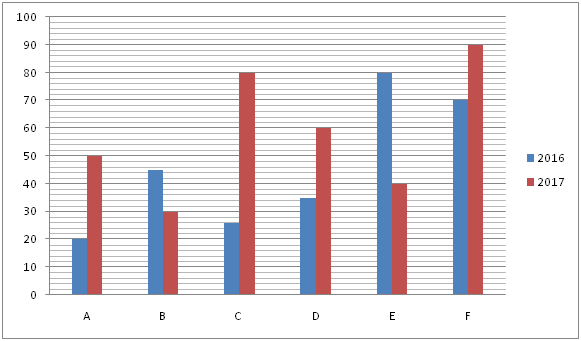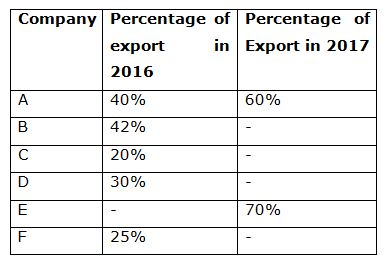# IBPS Clerk Mains Quantitative Aptitude Questions 2019 – (Day-4)

Dear Aspirants, Our IBPS Guide team is providing new pattern Quantitative Aptitude Questions for IBPS Clerk Mains 2019 so the aspirants can practice it on a daily basis. These questions are framed by our skilled experts after understanding your needs thoroughly. Aspirants can practice these new series questions daily to familiarize with the exact exam pattern and make your preparation effective.

Check here for IBPS Clerk Prelims Mock Test 2019

[WpProQuiz 7354]

Data interpretation

Directions (1 – 5): Study the following information carefully and answer the given questions:

The following bar graph shows the production of mobiles (In thousands) of different companies in two different years.1) The average number of mobiles exported by company D and F in 2017 is 35000 while the mobiles exported by company D in 2016 is twice the export in 2017 then find the percentage of export by company F out of total production of company F in 2017?

a) 65 %

b) 72 %

c) 80 %

d) 85 %

e) 92 %

2) If company E exported a total of 50000 mobiles in the year 2016 and 2017, then find the percentage of mobiles exported by company E in 2016?

a) 35 %

b) 27.5 %

c) 20 %

d) 17.5 %

e) None of these

3) The total number of mobiles exported by company A in 2016 and 2017 is 2/3 of the mobiles exported by company C in these years then find the percentage of mobiles that were exported by company C out of total production in 2017 ?

a) 72 %

b) 56.25 %

c) 45 %

d) 64.75 %

e) 75 %

4) The 20% of the mobiles exported by company B in 2016 are defective while the ratio between the defective mobiles exported by company B in the year 2016 and 2017 is 7 : 5 and the percentage of defective mobiles out of total export by company B in 2017 is 13.5% then find the percentage of mobile exported by company B out of total production?

a) 45.66 %

b) 72.77 %

c) 48.55 %

d) 66.66 %

e) 56.33 %

5) Find the difference between the mobiles exported by company A, B and D in 2016 to that of the mobiles exported by company A and E in 2017?

a) 18500

b) 24200

c) 20600

d) 19700

e) None of these

Inequality

Directions (6 – 10): In the following questions, two quantities are given as Quantity I and Quantity II. By finding these quantities gives corresponding answer.

6) Quantity I: ‘x’: 4x2+24x= -27

Quantity II: ‘y’: 4y2+33y+35=0

a) Quantity I > Quantity II

b) Quantity I < Quantity II

c) Quantity I ≥ Quantity II

d) Quantity I ≤ Quantity II

e) Quantity I = Quantity II or No relation

7) Quantity I: A circle and a rectangle have the same area. Length and breadth of the rectangle is 77cm and 50cm respectively. What is the circumference of circle?

Quantity II: Diameter of a circle is 56 cm and perimeter of a rectangle is same as circumference of the circle. Find out the area of the rectangle, if the length of the rectangle is three times the breadth of the rectangle.

a) Quantity II > Quantity I

b) Quantity II < Quantity I

c) Quantity I ≥ Quantity II

d) Quantity I ≤ Quantity II

e) Quantity I = Quantity II or No relation

8) Quantity I: ‘X’: 5, 9, 36, X, 404, 525

Quantity II: ‘Y’: 11, 20, Y, 224, 1115, 6684

a) Quantity II > Quantity I

b) Quantity II < Quantity I

c) Quantity I ≥ Quantity II

d) Quantity I ≤ Quantity II

e) Quantity I = Quantity II or No relation

9) Quantity I: ‘X’:  The cost of 5 bags and 12 notes is Rs. 3100 and the cost of 7 bags and 18 notes is Rs. 4400. What is the cost of 3 notes?

Quantity II: ‘Y’:  Y2= 22500

a) Quantity II> Quantity I

b) Quantity II < Quantity I

c) Quantity I ≥ Quantity II

d) Quantity I ≤ Quantity II

e) Quantity I = Quantity II or No relation

10) Quantity I: “A”: X, Y, and Z can finish a work in 5, 6, and 10 Days respectively. They start working together. If Y stops after 2 days, how long would it take X and Z to finish the remaining work.

Quantity II: “B”: B3= 27/1331

a) Quantity II> Quantity I

b) Quantity II< Quantity I

c) Quantity I ≥ Quantity II

d) Quantity I ≤ Quantity II

e) Quantity I = Quantity II or No relation

Directions (1-5) :

Total mobiles exported by company D and F= 35000*2 = 70000

Mobile exported by company D in 2016= (30/100)*35000 = 10500

Mobile exported by company D in 2017 = 10500/2 = 5250

Mobile exported by company F = 70000 – 5250 = 64750

Percentage of production exported by company F in 2017

= > 64750/90000* 100 = 72%

Mobile exported by company E in 2017 = 40000*(70/100) = 28000

Mobile exported by company E in 2016 = 50000 – 28000 = 22000

Required % = (22000/80000)*100 = 27.5 %

Total number of mobiles exported by company A in 2016 and 2017

= > (40/100)*20000 + (60/100)*50000

= > 8000 + 30000 = 38000

Mobile exported by company C in 2016 and 2017

= > 38000*(3/2) = 57000

Mobile exported by company C in 2017

= > 57000 – 26000*(20/100)

= > 51800

Percentage of export by company C = (51800/80000)*100 = 64.75 %

Number of defective mobile exported by B in 2016

= > 45000*(42/100)*(20/100) = 3780

Number of defective mobile exported by company B in 2017

= > (3780/7)*5 = 2700

Number of mobiles exported by company B

= > (2700/13.5)*100 = 20000

Percentage of mobile exported by company B in 2017

= > (20000/30000)*100 = 66.66 %

Mobile exported by A, B and D in 2016

= > 20000*(40/100) + 45000*(42/100) + 35000*(30/100)

= > 8000 + 18900 + 10500 = 37400

Mobile exported by company A and E in 2017

= > 50000*(60/100) + 40000*(40/100)

= > 30000 + 28000 = 58000

Required difference = 58000 – 37400 = 20600

Directions (6-10) :

Quantity I:

4x2+24x= -27

4x2+24x+27=0

4x2+18x+6x+27=0

2x(2x+9)+3(2x+9)=0

X= – 3/2, – 9/2 = -1.5, -4.5

Quantity II:

4y2+33y+35=0

4y2+28y+5y+35=0

4y(y+7)+5(y+7)=0

y= – 5/4, – 7 = -1.2, -7

No Relation

Quantity I:

Area of circle= Area of Rectangle

πr2= (l × b)

22/7× r2= 77×50

r2= 25×49

r=35 cm

Circumference of circle= 2πr

= 2 × 22/7 × 35

= 220 cm

Quantity II:

Perimeter of rectangle= Circumference of circle

2(l + b) = 2πr

2(l + b) = 2 × 22/7 × 56/2

l + b= 88cm

According to the question,

3b+b= 88

b=22

Area of rectangle= l × b = 22×66= 1452cm2

Quantity II> Quantity I

Quantity I:

5, 9, 36, X, 404, 525

5 + 2= 9

9 + 33 = 36

36 + 561

61 + 73 = 404

404 + 112 = 525

Quantity II:

11, 20, Y, 224, 1115, 6684

11 * 2 – 2 = 20

20 * 3 – 3 = 57

57 * 4 – 4 = 224

224 * 5 – 5 = 1115

1115 * 6 – 6 = 6684

Quantity II < Quantity I

Quantity I:

According to the question:

5b + 12n = 3100—– (i)

7b + 18n = 4400 —– (ii)

After solving equation (i) and (ii),

We get, b= 500 and n= 50

Cost of 3 notes = 50×3= 150

Quantity II:

Y2= 22500

Y= ±150

Quantity I ≥ Quantity II

Quantity I:

According to the question,

X + Y + Z= 1/5 + 1/6 + 1/10

= (12+10+6)/60 = 28/60 = 7/15

Their work for 2 Days= 14/15

Remaining work = 1 – 14/15 = 1/15

Required Time to finish the work by X and Z

= (1/15)/(1/5 + 1/10) = 1/15 × 10/3 = 2/9 Days

Quantity II:

B3 = 27/1331 => B =3/11

Quantity II > Quantity I